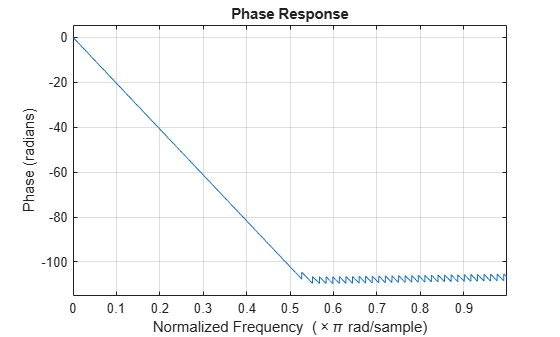# phasez

Phase response of discrete-time filter System object (unwrapped)

## Syntax

``````[phi,w] = phasez(sysobj)``````
``````[phi,w] = phasez(sysobj,n)``````
``````[phi,w] = phasez(___,'Arithmetic',arithType)``````
``phasez(sysobj)``

## Description

``````[phi,w] = phasez(sysobj)``` returns the unwrapped phase response `phi` of the filter System object™, `sysobj`, based on the current filter coefficients. The vector `w` contains the frequencies (in radians) at which the function evaluates the phase response. The phase response is evaluated at 8192 points equally spaced around the upper half of the unit circle.```
``````[phi,w] = phasez(sysobj,n)``` returns the phase response of the filter System object and the corresponding frequencies at `n` points equally spaced around the upper half of the unit circle.```
``````[phi,w] = phasez(___,'Arithmetic',arithType)``` analyzes the filter System object, based on the arithmetic specified in `arithType`, using either of the previous syntaxes.```

example

````phasez(sysobj)` displays the phase response of the filter System object `sysobj` in the `fvtool`.For more input options, see `phasez` in Signal Processing Toolbox™.```

## Examples

collapse all

```Fs = 8000; Fcutoff = 2000; FIRFilt = dsp.FIRFilter('Numerator', fir1(130,Fcutoff/(Fs/2)));```

`phasez` computes the unwrapped phase response of the filter and displays it using fvtool

`phasez(FIRFilt);`## Input Arguments

collapse all

Number of points over which the frequency response is computed. For an FIR filter where `n` is a power of two, the computation is done faster using FFTs.

Data Types: `single` | `double` | `int8` | `int16` | `int32` | `int64` | `uint8` | `uint16` | `uint32` | `uint64`

Arithmetic used in the filter analysis, specified as `'double'`, `'single'`, or `'Fixed'`. When the arithmetic input is not specified and the filter System object is unlocked, the analysis tool assumes a double-precision filter. When the arithmetic input is not specified and the System object is locked, the function performs the analysis based on the data type of the locked input.

The `'Fixed'` value applies to filter System objects with fixed-point properties only.

When the `'Arithmetic'` input argument is specified as `'Fixed'` and the filter object has the data type of the coefficients set to `'Same word length as input'`, the arithmetic analysis depends on whether the System object is unlocked or locked.

• unlocked –– The analysis object function cannot determine the coefficients data type. The function assumes that the coefficients data type is signed, has a 16-bit word length, and is auto scaled. The function performs fixed-point analysis based on this assumption.

• locked –– When the input data type is `'double'` or `'single'`, the analysis object function cannot determine the coefficients data type. The function assumes that the data type of the coefficients is signed, has a 16-bit word length, and is auto scaled. The function performs fixed-point analysis based on this assumption.

To check if the System object is locked or unlocked, use the `isLocked` function.

When the arithmetic input is specified as `'Fixed'` and the filter object has the data type of the coefficients set to a custom numeric type, the object function performs fixed-point analysis based on the custom numeric data type.

## Output Arguments

collapse all

Phase response vector of length `n`. If `n` is not specified, the function uses a default value of `8192`. The phase response is evaluated at `n` points equally spaced around the upper half of the unit circle.

Frequency vector of length `n`, in radians/sample. `w` consists of `n` points equally spaced around the upper half of the unit circle (from 0 to π radians/sample). If `n` is not specified, the function uses a default value of 8192.

### Topics

Introduced in R2011a

## SupportGet trial now